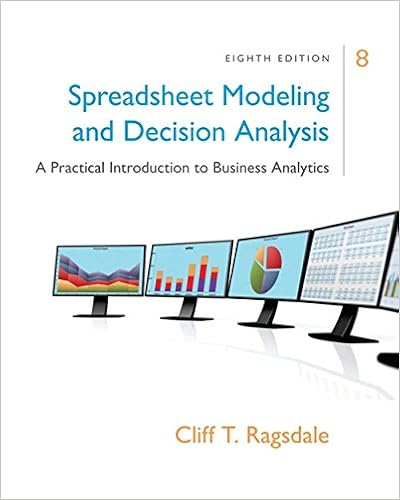# First consider the problem of optimizing y given x

• 134

This preview shows page 101 - 104 out of 134 pages.

##### We have textbook solutions for you!
The document you are viewing contains questions related to this textbook.The document you are viewing contains questions related to this textbook.
Chapter 2 / Exercise 2
RagsdaleExpert Verified
First, consider the problem of optimizingygivenx. This amounts to alinear program:minimizey(Px)subject toeTy= 1y0.It is easy to see that the basic feasible solutions of this linear program aregiven bye1, . . . , eM, where eacheiis the vector with all components equal to0 except for theith, which is equal to 1. It follows thatmin{y2<M|ye=1,y0}yPx=mini2{1,...,M}(Px)i.This minimal value can also be expressed as the solution to a linear program:maximizevsubject tovePx,wherev2 <is the only decision variable andxis fixed. In particular, theoptimal valuevresulting from this linear program satisfiesv=min{y2<M|ye=1,y0}yPx.To determine an optimal strategy for player 1, we find the value ofxthatmaximizesv. In particular, an optimal strategy is delivered by the followinglinear program:maximizevsubject tovePxex= 1x0,wherev2 <andx2 <Nare decision variables. An optimal solution to thislinear program provides a stochastic strategyxthat maximizes the payov,
##### We have textbook solutions for you!
The document you are viewing contains questions related to this textbook.The document you are viewing contains questions related to this textbook.
Chapter 2 / Exercise 2Expert Verified
•••